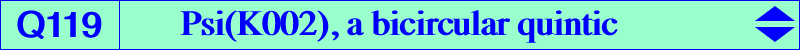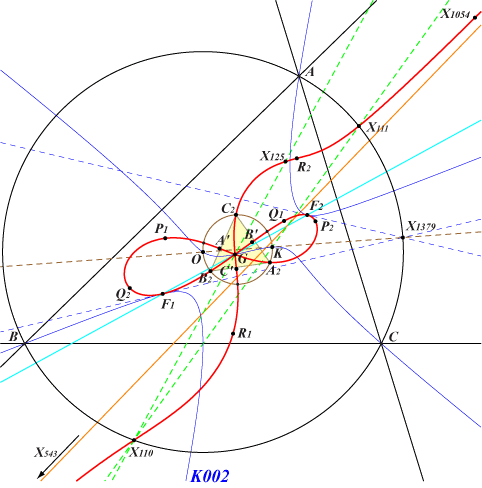too complicated to be written here. Click on the link to download a text file.X(2), X(110), X(111), X(125), X(543), X(1054) foci of the Steiner inellipse other points belowQ119 is the Psi transform of the Thomson cubic K002. See the analogous Q041, Q048. Hence, it contains the singular points of Psi (X2 which is a triple point on the curve, the circular points at infinity which are double points), the Psi-images of all the points of K002, for example : • X(110), X(111), X(125), X(1054) which are the images of X(3), X(6), X(4), X(1) respectively. • the foci of the Steiner inellipse which are invariant under Psi. Note that the tangents at the real foci F1, F2 are the same for K002, Q119 and concur at X(1379) on the circumcircle and on the Brocard axis. Similarly, the tangents at the imaginary foci pass through X(1380). • A2, B2, C2, the vertices of the second Brocard triangle which are the Psi-images of A, B, C. The tangents at these points concur at : a^2 (a^4-a^2 b^2+4 b^4-a^2 c^2-16 b^2 c^2+4 c^4) : : , on the lines {2,5503}, {6,373}, {51,11173}, {111,182}, etc, SEARCH = 7.61080086263007. This point is now X(22111) in ETC (2018-09-04). The three remaining points on the Brocard circle are the Psi-images of the vertices of the Thomson triangle. The tangents at these points also concur at : a^2 (a^4-a^2 b^2-a^2 c^2-8 b^2 c^2) : : , on the lines {2,98}, {3,373}, {6,5646}, {22,6688}, {51,1350}, etc, SEARCH = 2.91139235655761. This point is now X(22112) in ETC (2018-09-04). • A', B', C', Psi-images of the excenters and extraversions of X(1054). The tangents at X(1054) and at these points concur at X(2). • P1, Q1, R1, Psi-images of the midpoints of ABC, on the corresponding McCay circles. • P2, Q2, R2, Psi-images of the midpoints of the altitudes of ABC. P2 lies on the circle passing through X(2), X(125), A2.